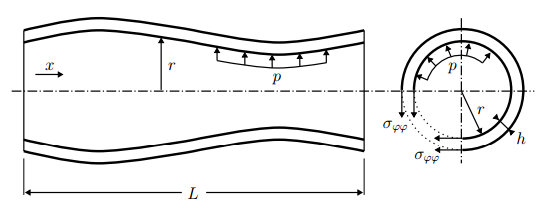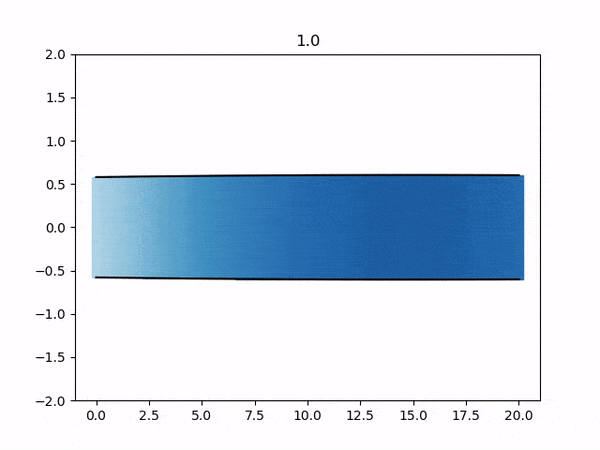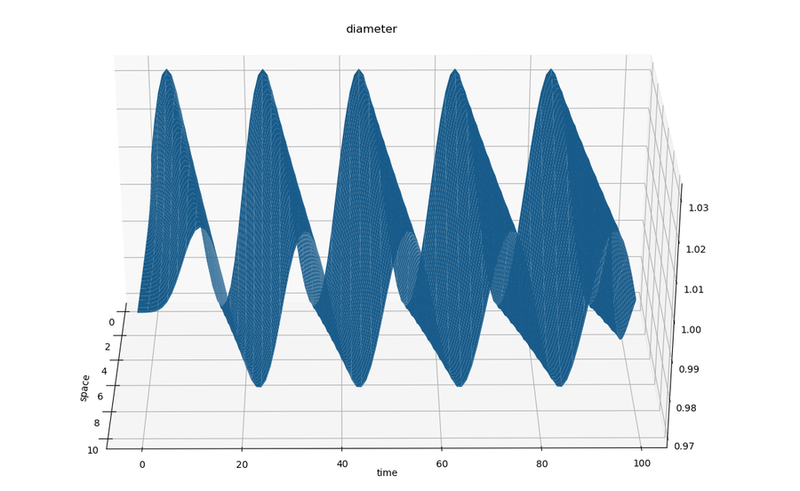The 1D Elastic Tube is a FSI case, that consists of an internal flow in a flexible tube. The flow is unsteady and incompressible. This tutorial contains C++ and Python variants of the fluid and solid solvers. Running the simulation takes just 1-2 minutes.

## Setup

We want to simulate the internal flow in a flexible tube as shown in the figure below (image from ).The flow is assumed to be incompressible flow and gravity is neglected. Due to the axisymmetry, the flow can be described using a quasi-two-dimensional continuity and momentum equations. The motivation and exact formulation of the equations that we consider can be found in .

The following parameters have been chosen:

• Length of the tube: L = 10
• Inlet velocity: $$v_{inlet} = 10 + 3 sin (10 \pi t)$$
• Initial cross sectional area = 1
• Initial velocity: v = 10
• Initial pressure: p = 0
• Fluid density: $$\rho = 1$$
• Young modulus: E = 10000

Additionally the solvers use the parameters N = 100 (number of cells), tau = 0.01 (dimensionless timestep size), kappa = 100 (dimensionless structural stiffness) by default. These values can be modified directly in each solver.

## Available solvers

Both fluid and solid participant are supported in:

• C++: An example solver using the intrinsic C++ API of preCICE. This solver also depends on LAPACK (e.g. on Ubuntu sudo apt-get install liblapack-dev)
• Python: An example solver using the preCICE Python bindings. This solver also depends on the Python libraries numpy scipy matplotlib vtk mpi4py, which you can get from your system package manager or with pip3 install --user <package>.

### Building the C++ Solver

In order to use the C++ solver, you first need to build the scripts FluidSolver and SolidSolver. Each script needs to be built separately.

cd fluid-cpp
mkdir build && cd build
cmake ..
make all

cd solid-cpp
mkdir build && cd build
cmake ..
make all


Building can be skipped if you do not plan to use the C++ version.

## Running the Simulation

### C++

Open two separate terminals and start each participant by calling the respective run script.

cd fluid-cpp
./run.sh


and

cd solid-cpp
./run.sh


### Python

Open two separate terminals and start each participant by calling the respective run script. Only serial run is possible:

cd fluid-python
./run.sh


and

cd solid-python
./run.sh


Optional: A run-time plot visualization can be triggered by passing --enable-plot in run.sh of FluidSolver.py. Additionally a video of the run-time plot visualization can be generated by additionally passing --write-video

## Post-processingThe results from each simulation are stored in each fluid-<participant>/output/ folder. You can visualize these VTK files using the provided plot-diameter.sh script

./plot-diameter.sh


which will try to visualize the results from both fluid cases, if available. This script calls the more flexible plot-vtk.py Python script, which you can use as

python3 plot-vtk.py <quantity> <case>/output/<prefix>


Note the required arguments specifying which quantity to plot (pressure, velocity or diameter) and the name prefix of the target vtk files.

For example, to plot the diameter of the fluid-python case using the default prefix for VTK files, plot-diameter.sh executes:

python3 plot-vtk.py diameter fluid-python/output/out_fluid_B. Gatzhammer. Efficient and Flexible Partitioned Simulation of Fluid-Structure Interactions. Technische Universitaet Muenchen, Fakultaet fuer Informatik, 2014.

 J. Degroote, P. Bruggeman, R. Haelterman, and J. Vierendeels. Stability of a coupling technique for partitioned solvers in FSI applications. Computers & Structures, 2008.

 M. Mehl, B. Uekermann, H. Bijl, D. Blom, B. Gatzhammer, and A. van Zuijlen. Parallel coupling numerics for partitioned fluid-structure interaction simulations. CAMWA, 2016.